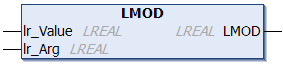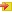# LMODThe LMOD function carries out a modulo division and returns the signed divide remainder.

Examples

LMOD(400.56, 360) = 40.56

LMOD(-400.56, 360) = -40.56

Similar functions: MOD, MODABSUnlike MOD, the LMOD function operates with floating point variables and also determines non-integer remainders.

In the context of NC axes, modulo values are usually used unsigned. These can be calculated with the MODABS function.

##Inputs

`VAR_INPUT    lr_Value : LREAL;    lr_Arg   : LREAL;END_VAR`

Name

Type

Description

lr_Value

LREAL

Input value

lr_Arg

LREAL

Modulo range

## Requirements

Development environment

Target system type

PLC libraries to include

TwinCAT v3.0.0

PC or CX (x86)

Tc2_Math